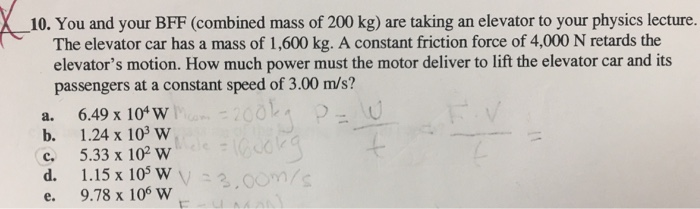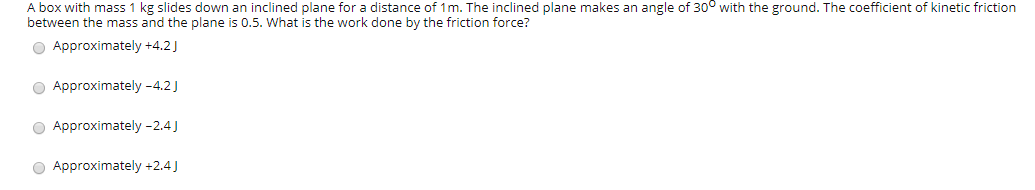QuestionSolution : the power of the system is given by the equation as

Power = Force x velocity

Here force required in upward direction = weight of the system + friction force = 1800x9.81 + 4000 = 21658 N

Now power= 21658 x 3 = 64974 watt = 6.49 x 104 watt

#### Earn Coins

Coins can be redeemed for fabulous gifts.

Similar Homework Help Questions
• ### Use the worked example above to help you solve this problem. A 1.19 103-kg elevator car...

Use the worked example above to help you solve this problem. A 1.19 103-kg elevator car carries a maximum load of 7.40 102 kg. A constant friction force of 4.07 103 N retards its motion upward, as shown in the figure. What minimum power, in kilowatts and horsepower, must the motor deliver to lift the fully loaded elevator at a constant speed of 3.00 m/s? P = kW P = hp

• ### I need all 3 questions please A box with mass 1 kg slides down an inclined...I need all 3 questions please A box with mass 1 kg slides down an inclined plane for a distance of 1m. The inclined plane makes an angle of 30° with the ground. The coefficient of kinetic friction between the mass and the plane is 0.5. What is the work done by the friction force? Approximately +4.2) Approximately -4.2) Approximately -2.4) Approximately +2.4) From the choices given below. choose the correct magnitude and direction of the center of mass of...